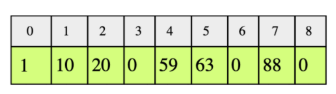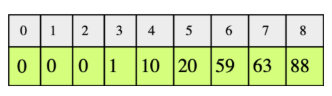# Move Zeros to the Left

## Problem Statement

Given an integer array, move all elements that are 0 to the left while maintaining the order of other elements in the array. The array has to be modified in-place.

Let’s look at the following integer array:After moving all zeros to the left, the array should like this:Remember to maintain the order of non-zero elements

## Hint

• Use the concept of reader/writer indexes

## Try it yourself

void move_zeros_to_left(int A[], int n) {  //TODO: Write - Your - Code}

## Solution

void move_zeros_to_left(int A[], int n) {     if (n < 1) return;    int write_index = n - 1;  int read_index = n - 1;  while(read_index >= 0) {    if(A[read_index] != 0) {      A[write_index] = A[read_index];      write_index--;    }    read_index--;  }  while(write_index >= 0) {    A[write_index] = 0;    write_index--;  }}int main() {  int v[] = {1, 10, 20, 0, 59, 63, 0, 88, 0};  int n = sizeof(v) / sizeof(v);  cout << "Original Array" <<endl;    for(int x=0 ; x<n; x++) {    cout << v[x];    cout << ", ";  }      move_zeros_to_left(v, n);    cout << endl<< "After Moving Zeroes to Left"<< endl;  for(int i=0 ; i<n; i++) {    cout << v[i];    cout << ", ";  }  }

## Solution Explanation

### Runtime complexity

The runtime complexity if this solution is linear, O(n).

### Memory complexity

The memory complexity of this solution is constant, O(1).

### Breakdown of solution

Keep two markers: read_index and write_index and point them to the end of the array. Let’s take a look at an overview of the algorithm:

While moving read_index towards the start of the array:

• If read_index points to 0, skip.

• If read_index points to a non-zero value, write the value at read_index to write_index and decrement write_index.

• Assign zeros to all the values before the write_index and to the current position of write_index as well.

Practice problems like this and many more by checking out our Grokking the Coding Interview: Patterns for Coding Questions course!

Learn in-demand tech skills in half the time